# Program to check Twin prime In Java

In this tutorial you will learn about the Program to check Twin prime In Java and its application with practical example.

In this tutorial, we will learn to create a Java Program to check Twin prime In Java using Java programming.

## Prerequisites

Before starting with this tutorial we assume that you are best aware of the following Java programming topics:

• Java Operators.
• Basic Input and Output function in Java.
• Class and Object in Java.
• Basic Java programming.
• If-else statements in Java.
• For loop in Java.

## What is Twin Prime Number.

A Number is called a Prime if it is Divisible by itself and Twin Prime number having a prime gap of two(2) is referred to as a twin prime number.

For Example:->

1. 29 and 31 are Twin Prime Number.(31-29=2).
2. 3 and 5 are Twin Prime Number.(5-3 =2).
3. 71 and 73 are Twin Prime Number.(73-71 =2).

## Program to check Twin prime In Java

In this program we would find given numbers are Twin prime number or not .first of we would take a value from user and find the Twin prime .let have a look at the code

## Output

Twin Prime number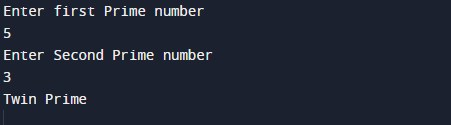Not a Twin Prime number.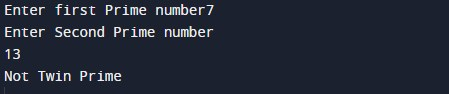In the above program, we have first declared and initialized a set variables required in the program.

• number = it will hold entered numbers
• num,num1,no1 and no2=   it will hold entered numbers.

After that we take a number from user and find given number is a Twin Prime number or not.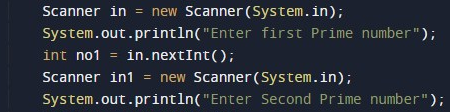After taking two prime number we pas this number to Prime() function where we calculate is there difference or prime number is 2 if yes.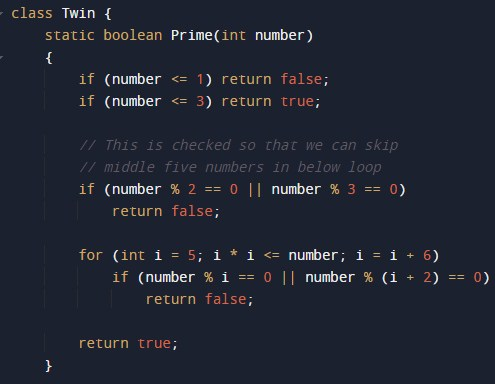Function returns true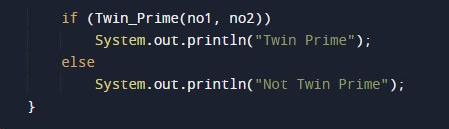else return False.

In this tutorial we have learn about the Program to check Twin prime In Java and its application with practical example. I hope you will like this tutorial.